# How to Join Pandas DataFrames using Merge?

• Difficulty Level : Expert
• Last Updated : 05 May, 2022

Joining and merging DataFrames is that the core process to start  out with data analysis and machine learning tasks. It’s one of the toolkits which each Data Analyst or Data Scientist should master because in most cases data comes from multiple sources and files. In this tutorial, you’ll how to join data frames in pandas using the merge technique. More specifically, we will practice the concatenation of DataFrames along row and column.

## Getting Started

The most widely used operation related to DataFrames is the merging operation. Two DataFrames might hold different kinds of information about the same entity and they may have some same columns, so we need to combine the two data frames in pandas for better reliability code. To join these DataFrames, pandas provides various functions like join(), concat(), merge(), etc. In this section, you will practice using the merge() function of pandas. There are basically four methods of merging:

• inner join
• outer join
• right join
• left join

### Inner join

From the name itself, it is clear enough that the inner join keeps rows where the merge “on” value exists in both the left and right dataframes.Now let us create two dataframes and then try merging them using inner.

## Python3

 `import` `numpy as np``import` `pandas as pd`  `left ``=` `pd.DataFrame({``'Sr.no'``: [``'1'``, ``'2'``, ``'3'``, ``'4'``, ``'5'``],``                    ``'Name'``: [``'Rashmi'``, ``'Arun'``, ``'John'``,``                             ``'Kshitu'``, ``'Bresha'``],``                    ``'Roll No'``: [``'1'``, ``'2'``, ``'3'``, ``'4'``, ``'5'``]})` `right ``=` `pd.DataFrame({``'Sr.no'``: [``'2'``, ``'4'``, ``'6'``, ``'7'``, ``'8'``],``                    ``'Gender'``: [``'F'``, ``'M'``, ``'M'``, ``'F'``, ``'F'``],``                    ``'Interest'``: [``'Writing'``, ``'Cricket'``, ``'Dancing'``,``                                 ``'Chess'``, ``'Sleeping'``]})``                        ` `# Merging the dataframes                    ``pd.merge(left, right, how ``=``'inner'``, on ``=``'Sr.no'``)`

Output: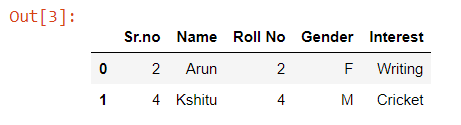### Outer join

An outer join returns all the rows from the left dataframe, all the rows from the right dataframe, and matches up rows where possible, with NaNs elsewhere. But if the dataframe is complete, then we get the same output.## Python3

 `import` `numpy as np``import` `pandas as pd`  `left ``=` `pd.DataFrame({``'Sr.no'``: [``'1'``, ``'2'``, ``'3'``, ``'4'``, ``'5'``],``                    ``'Name'``: [``'Rashmi'``, ``'Arun'``, ``'John'``,``                             ``'Kshitu'``, ``'Bresha'``],``                    ``'Roll No'``: [``'1'``, ``'2'``, ``'3'``, ``'4'``, ``'5'``]})` `right ``=` `pd.DataFrame({``'Sr.no'``: [``'2'``, ``'4'``, ``'6'``, ``'7'``, ``'8'``],``                    ``'Gender'``: [``'F'``, ``'M'``, ``'M'``, ``'F'``, ``'F'``],``                    ``'Interest'``: [``'Writing'``, ``'Cricket'``, ``'Dancing'``,``                                 ``'Chess'``, ``'Sleeping'``]})``                        ` `# Merging the dataframes                    ``pd.merge(left, right, how ``=``'outer'``, on ``=``'Sr.no'``)`

Output: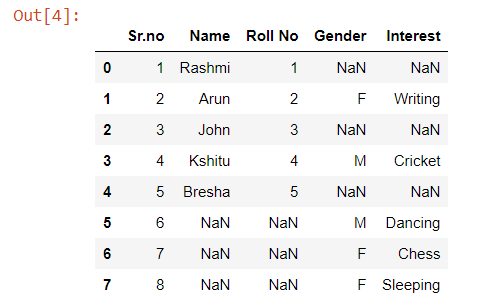### Left join

With a left join, all the records from the first dataframe will be displayed, irrespective of whether the keys in the first dataframe can be found in the second dataframe. Whereas, for the second dataframe, only the records with the keys in the second dataframe that can be found in the first dataframe will be displayed.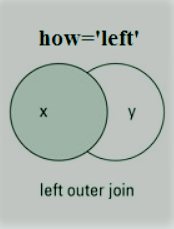## Python3

 `import` `numpy as np``import` `pandas as pd`  `left ``=` `pd.DataFrame({``'Sr.no'``: [``'1'``, ``'2'``, ``'3'``, ``'4'``, ``'5'``],``                    ``'Name'``: [``'Rashmi'``, ``'Arun'``, ``'John'``,``                             ``'Kshitu'``, ``'Bresha'``],``                    ``'Roll No'``: [``'1'``, ``'2'``, ``'3'``, ``'4'``, ``'5'``]})` `right ``=` `pd.DataFrame({``'Sr.no'``: [``'2'``, ``'4'``, ``'6'``, ``'7'``, ``'8'``],``                    ``'Gender'``: [``'F'``, ``'M'``, ``'M'``, ``'F'``, ``'F'``],``                    ``'Interest'``: [``'Writing'``, ``'Cricket'``,``                                 ``'Dancing'``, ``'Chess'``,``                                 ``'Sleeping'``]})``                        ` `# Merging the dataframes                    ``pd.merge(left, right, how ``=``'left'``, on ``=``'Sr.no'``)`

Output: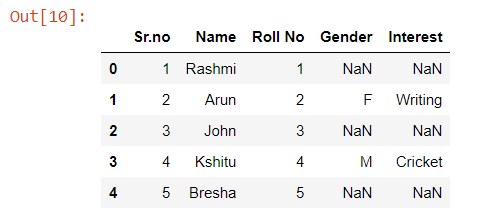Note the Output Carefully.

### Right join

For a right join, all the records from the second dataframe will be displayed. However, only the records with the keys in the first dataframe that can be found in the second dataframe will be displayed.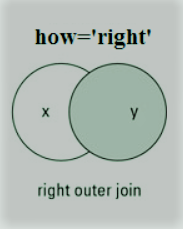## Python3

 `import` `numpy as np``import` `pandas as pd`  `left ``=` `pd.DataFrame({``'Sr.no'``: [``'1'``, ``'2'``, ``'3'``, ``'4'``, ``'5'``],``                    ``'Name'``: [``'Rashmi'``, ``'Arun'``, ``'John'``,``                             ``'Kshitu'``, ``'Bresha'``],``                    ``'Roll No'``: [``'1'``, ``'2'``, ``'3'``, ``'4'``, ``'5'``]})` `right ``=` `pd.DataFrame({``'Sr.no'``: [``'2'``, ``'4'``, ``'6'``, ``'7'``, ``'8'``],``                    ``'Gender'``: [``'F'``, ``'M'``, ``'M'``, ``'F'``, ``'F'``],``                    ``'Interest'``: [``'Writing'``, ``'Cricket'``, ``'Dancing'``,``                                 ``'Chess'``, ``'Sleeping'``]})``                        ` `# Merging the dataframes                    ``pd.merge(left, right, how ``=``'right'``, on ``=``'Sr.no'``)`

Output: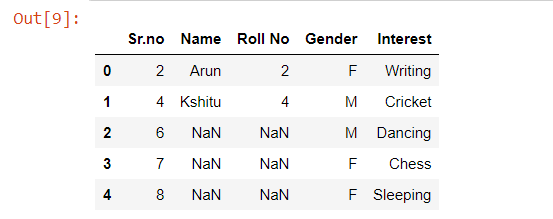My Personal Notes arrow_drop_up